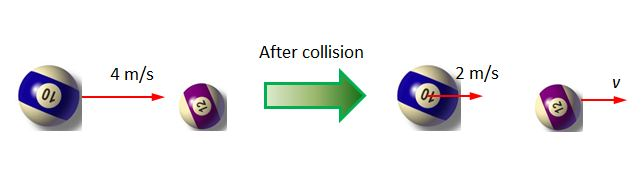# Collision of billiard ballsThe masses of the blue ball (#$10$) and the purple ball (#$12$) are $2$ kg and $1$ kg, respectively. Now, the blue ball with a velocity of $4$ m/s collides with the purple ball which was standing still. After the collision, the blue ball keeps moving in the same direction with a reduced velocity of $2$ m/s. What is the velocity of the purple ball after the collision?

×